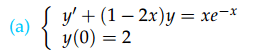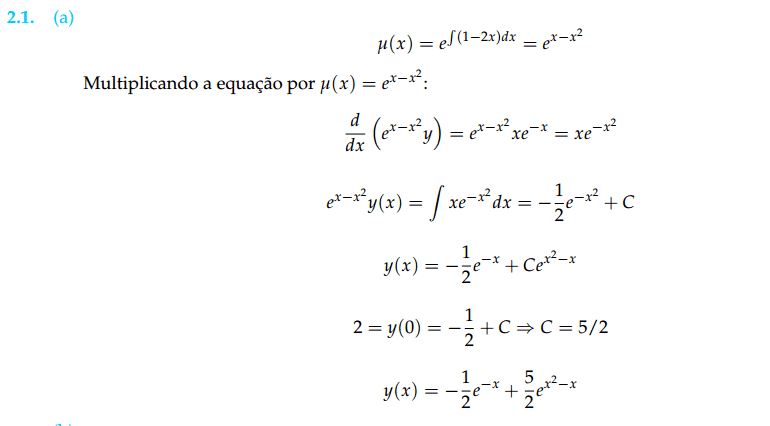# Linear Differential Equation - Initial Value Problem

• thaalescosta

#### thaalescosta

Hello, I'm struggling with a simple problem here.

It asks me to solve the following initial value problem:So far I've calculated the integration factor μ(x) = ex-x2 and I multiplied both sides of the equation by it and got this:
http://www4c.wolframalpha.com/Calculate/MSP/MSP36171f59839bdf0g6cbd000044defig6e40h0ei0?MSPStoreType=image/gif&s=64&w=256.&h=47. [Broken]

The problem I'm having is that the equation I got is different than the equation in the first line of the solution:I don't understand how the ex-x2⋅(1 - 2x)⋅y disappeared

EDIT: Actually, nevermind. My brain was too lazy to realize that the left part of the equation is equal to the derivative of ex-x2⋅y so I could just simplify it

Last edited by a moderator: